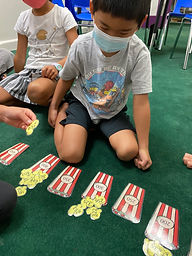## Ms. Brianne

### Target 1​

###### Lesson Type:

Continuation

Number Operation

:

Integer Composition

Use place-value understandings to round whole numbers to the nearest ten or 100.

###### 1:

Use understanding of how to round two-digit numbers and expand the strategy to three-digit numbers.

###### 2:

Understand that rounding identifies the multiple of 10 or 100 that is closest to the given number.

###### 3:

Understand that the previous place (one place smaller) is the key digit to evaluate to determine whether a number is rounded up or down.

3rd

###### Vocabulary:

Rounding, Nearest Ten, Nearest Hundred

Activities:

• Students practiced rounding numbers to the nearest ten or hundred and sorted pictures of numbered popcorn according to the number it rounded to.
• Students used number cards to create their own 2 and 3-digit numbers. Then they rounded the numbers to the nearest ten or hundred. They were able to use a number line to help them visually see the nearest ten or hundred.### Home Exploration

###### Guiding Questions:## Absent Students:

### Target 2

:

###### 1:

Understand that a graph is a visual representation of data.

###### 2:

Become familiar with the vocabulary associated with graphs: unit, scale, title and labels.

###### 3:

Construct and or read a simple picture graph.

###### 4:

Construct and read a scaled bar graph.

3rd

###### Vocabulary:

Data, Quantity, Tally Marks, Bar Graph

Activities:

• Students took data and created graphs using skittles. First they sorted and filled in a frequency chart using tally marks. Then they used their data to create a picture graph with a key, and a bar graph that included a title, labels, units, and scale of their choice. Finally they used their graphs to answer questions about the data.
• Students answered questions by interpreting the data shown in a given bar graph.### Home Exploration

###### Guiding Questions:### Target 3

:

###### Vocabulary:

Activities:### Home Exploration